# Lattice Boltzmann Simulation of Magnethydrodynamics Natural Convection in an L-Shaped Enclosure

Lattice Boltzmann Simulation of Magnethydrodynamics Natural Convection in an L-Shaped Enclosure

Bouchmel MlikiMohamed Ammar Abbassi Ahmed Omri

Unité de Recherche Matériaux, Energie et Energies Renouvelables (MEER), Faculté des Sciences de Gafsa, B.P.19, Zarroug, Gafsa, 2112, Tunisie

Corresponding Author Email:
bouchmelmliki@hotmail.com
Page:
565-573
|
DOI:
https://doi.org/10.18280/ijht.340403
|
Accepted:
|
Published:
31 December 2016
| Citation

OPEN ACCESS

Abstract:

This paper presents the results of a numerical study on the natural convection of Al2O3-H2O nanofluid in an L-shaped cavity in the presence of a uniform magnetic field. The Lattice Boltzmann Method (LBM) is used to solve the governing equations. The effective thermal conductivity and viscosity of nanofluid are calculated by KKL (Koo-Kleinstreuer-Li) correlation. The influence of pertinent parameters such as Rayleigh number (104Ra≤106), Hartmann number (0≤Ha≤60), nanoparticle volume concentration (0≤f≤0.04) and the cavity aspect ratio (0.2≤AR ≤0.5) on the flow and heat transfer characteristics have been examined. The heat transfer increases first, then considering the role of Brownian motion of nanoparticles and increases with increasing of volume fraction of nanoparticles. But, on the contrary, Hartmann number and aspect ratio of the cavity have others important improvement factor for decreasing free convection in the enclosures.

Keywords:

Brownian motion, Heat transfer, L-shaped cavity, Lattice Boltzmann Method, Nanofluid.

1. Introduction

Natural convection flows generated by buoyancy forces in rectangular closed cavities filled by nanofluid have been the object of numerous studies in the past. Actually, considerable efforts are still devoted in the area, where the effect of the applied of a uniform magnetic field is studied because of its wide applications in different areas such as cooling of electronic devices cooling, chemical processing equipment and solar energy collectors [1-5].

Various investigations on natural convection in different geometric cavities were done by researchers with different numerical methods. Mliki et al.  analyzed the natural convection heat transfer in an L-shaped cavity filled with nanofluids, by using lattice Boltzmann method. They analyzed the effect of different parameters such as Rayleigh number (103≤Ra≤106), aspect ratio of the L-shaped enclosure (0.2≤AR≤0.6) and nanoparticle volume concentration (0.0≤f ≤0.05) on the flow and temperature fields. The presented results indicated the decrease in heat transfer rate with aspect ratio and linear variation of this rate with Rayleigh number. Also, the addition of nanoparticle increases heat transfer for different studied parameters. Elshehabey et al.  have performed a numerical study of natural convection of a nanofluid in an inclined L-shaped differentially heated cavity with an inclined magnetic field.  Transfer equations have been solved using an implicit finite difference scheme and success under relaxation SUR algorithm. They reported that nanofluid enhances the heat transfer amount and reducing the aspect ratio improves this effect. Also it was found that the average Nusselt number decreases by an increase in Hartmann number, especially for higher Rayleigh numbers. Mliki et al.  studied the Cu/water nanofluid double-diffusive natural convection in a C-shaped cavity. They showed that heat and mass transfers decrease with increasing the Hartmann number. Also, increasing the Lewis number will enhance the mass transfer but reduces the heat transfer rate. Chen and Cheng  applied the finite-volume method and curvilinear coordinates to investigate buoyancy driven heat transfer enhancement in an inclined arc-shaped enclosure. They reported that the arc-shaped enclosure for Pr=4.0 at Gr= 4×106 and θ= π/2 exhibits the best heat transfer performance. Also it was found that the increase in natural convection heat transfer becomes appreciable when Gr>105. Mahmoodi and Hashemi  analyzed the free convection cooling in an L-shaped enclosure filled with copper-water nanofluid, by using finite volume approach. The presented results indicated that the nanofluid heat transfer rate increase with an increase in the Rayleigh number and volume fraction of Cu nanoparticles. Also, it was found that the rate of heat transfer is increased with decreasing the aspect ratio of the cavity. Free convection cooling in modified L-shaped enclosures filled with copper–water nanofluid, was studied numerically by Saidi and Karimi . In their study, the governing equations are solved numerically using finite volume approach. The effect of nanoparticle volume fraction on the enhancement of heat transfer has been investigated for several values sets of Rayleigh, the inclination angle and aspect ratio of the L-shaped enclosure. Their results indicated that the numbers of eddies increases with the increase of Rayleigh number. Also, it was found that the heat transfer increases by an increase in nanofluid particle concentration, for all ranges of Rayleigh number. In addition, the results indicate that the inclination angle is another important improvement factor for increasing free convection in the enclosures. Recently, Makulati et al.  studied the problem of MHD free convection in an inclined C-shaped enclosure. The governing equations are solved numerically using the finite volume formulation and the SIMPLE algorithm is used for pressure–velocity coupling. They showed that the Nusselt number has direct relationship with aspect ratio, nanoparticles volume fraction and inclination angle values while it has reverse relationship with Hartmann number. In another study, Mansour et al.  investigated numerically the natural convection in a T-shaped enclosures filled with Cu-Water nanofluid using finite difference method. The presented results showed that the average Nusselt number increased with increase in Rayleigh number and volume fraction of Cu nanoparticles regardless aspect ratio of the enclosure. Also, the results indicate that the effect of Cu nanoparticles on enhancement of heat transfer for narrow enclosures was more than that for wide enclosures.

The effect of nanoparticles Brownian motion is an important factor for the increasing the heat transfer inside a cavity field with nanofluid. Different studies have been adopted by different researchers when considering the role of Brownian motion of nanoparticles. Ghasemi and Aminossadati  have been numerically analyzed the effect of Brownian motion of nanoparticules on natural convection heat transfer and fluid flow in an enclosure filled with CuO-nanofluid. They found that the heat transfer of the nanofluid increases when considering the Brownian motion. In another study, Haddad et al.  investigated the natural convection in a square cavity heated from the bottom filled with CuO–water nanofluid. Results indicated that the enhancement in heat transfer is observed at any Rayleigh numbers and nanoparticle volume fraction by considering the role of Brownian motion. Recently, Mliki et al.  have performed a numerical study using the Lattice Boltzmann method in a linearly/sinusoidally heated cavity filled with a pure fluid or a nanofluid. In this study, the effective thermal conductivity and viscosity of nanofluid were calculated by the KKL (Koo-Kleinstreuer-Li) correlation. The presented results show that fluid flow and heat transfer characteristics are highly affected by the nanoparticles Brownian motion. In addition, the heat generation or absorption influences the heat transfer in the cavity at Ra = 103 more than other Raleigh numbers as the least effect is observed at Ra = 106.

The main aim of the present work is to discuss the effect of nanoparticles Brownian motion on heat transfer in a nanofluid-filled L-shaped enclosure with uniform boundary condition under the effect of magnetic field. The results will be presented in this work via streamlines, isotherms, average Nusselt number at different nanoparticle volume fractions, Hartman and Rayleigh numbers.

2. Problem Description

The geometry of the present study is an inclined L-shaped cavity filled with an Al2O3-H2O nanofluid under magnetic field B which is shown in figure 1. It consists of an L-shaped enclosure with H as height and W as width and the thickness is D. For the present case, the height and width are considered to be equal, H = W. The cavity aspect ratio is AR = H/D. The hot walls (ABC), the cold walls (DEF) and the adiabatic walls (AF and CD) are shown in this figure. The nanofluid is simulated as a single-phase homogeneous fluid, Newtonian, laminar and incompressible.

## 1.png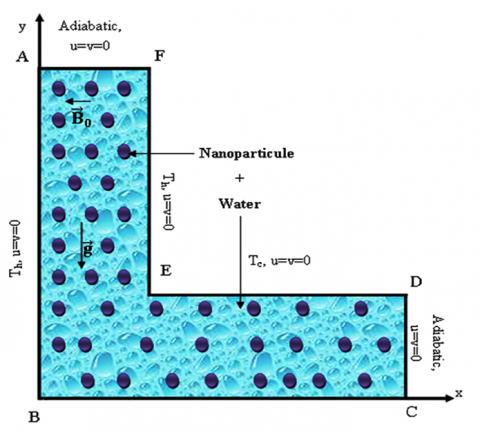Figure 1. Geometry of the problem

Thermophysical properties of the nanoparticles and the base liquid are gathered in Table 1. The density variation in the nanofluid is approximated by the standard Boussinesq approximation. Furthermore, it is assumed that the induced magnetic field produced by the motion of an electrically conducting fluid, viscous dissipation and Joule heating are neglected.

Table 1. Thermo-physical properties of water and nanoparticles

 Physical Properties H2O Al2O3 Cp(J/kg.K) 4179 765 r (kg/m3) 997.1 3970 k (W/m.K) 0.613 25 b´10-5 (1/K) 21 0.85 dp (nm) - 47 σ (Ω.m)-1 0.05 1.0 10-10
3. Mathematical Formulation

The continuity Eq. (1), the momentum Eqs. (2) and (3) and the energy Eq. (4) for MHD natural convection can be written in a non-dimensional form, respectively by

$\frac{\partial U}{\partial X}+\frac{\partial V}{\partial Y}=0$(1)

$U \frac{\partial U}{\partial X}+V \frac{\partial U}{\partial Y}=-\frac{\partial P}{\partial X}+\operatorname{Pr}\left(\frac{\partial^{2} U}{\partial X^{2}}+\frac{\partial^{2} U}{\partial Y^{2}}\right)+R a \operatorname{Pr} \theta \sin \gamma$(2)

$U \frac{\partial V}{\partial X}+V \frac{\partial V}{\partial Y}=-\frac{\partial P}{\partial Y}+\operatorname{Pr}\left(\frac{\partial^{2} V}{\partial X^{2}}+\frac{\partial^{2} V}{\partial Y^{2}}\right)+R a \operatorname{Pr} \theta \cos \gamma-H a^{2} \operatorname{Pr} V$(3)

$U \frac{\partial \theta}{\partial X}+V \frac{\partial \theta}{\partial Y}=\left(\frac{\partial^{2} \theta}{\partial X^{2}}+\frac{\partial^{2} \theta}{\partial Y^{2}}\right)$(4)

In these equations the non-dimensional parameters are defined by

$X=\frac{x}{H}, \quad \mathrm{Y}=\frac{y}{H}, \quad U=\frac{u H}{\alpha_{n f}}$,$V=\frac{v H}{\alpha_{n f}}, \quad \theta=\frac{T-T_{c}}{T_{h}-T_{c}}$,$P=\frac{p H^{2}}{\rho_{n f} \alpha_{n f}^{2}}$,$\operatorname{Pr}=\frac{v_{n f}}{\alpha_{n f}}$,$R a=\frac{g \beta\left(\mathrm{T}-\mathrm{T}_{c}\right) H^{3}}{v_{n f} \alpha_{n f}}$,$H a=H B \sqrt{\frac{\sigma_{n f}}{\mu_{n f}}}$(5)

The effective density (ρnf), the thermal expansion coefficient (βnf), heat capacitance (ρCp)nf  and thermal diffusivity of the nanofluid are respectively defined by:In these definitions σnf is electrical conductivity of nanofluid and B is the magnitude of the magnetic field.

$\rho_{n f}=(1-\phi) \rho_{f}+\phi \rho_{p}$(6)

$\left(\rho C_{p}\right)_{n f}=(1-\phi)\left(\rho C_{p}\right)_{f}+\phi\left(\rho C_{p}\right)_{p}$(7)

$(\rho \beta)_{n f}=(1-\phi)(\rho \beta)_{f}+\phi(\rho \beta)_{p}$(8)

$\alpha_{n f}=\frac{k_{n f}}{\left(\rho C_{p}\right)_{n f}}$(9)

For thermal conductivity, we used the model developed by Koo and Kleinstreuer  that considers the nanoparticle Brownian motion. This model takes into account the effects of particle volume fraction, particle size and nanofluid temperature.

$k_{e f f}=k_{s t a t i c}+k_{B r o w n i a n}$(10)

$k_{\text {static}}$ is the static thermal conductivity based on Maxwell classical correlation and$k_{\text {Brownian}}$ is the component considered the enhanced thermal conductivity by micro-scale convective heat transfer of a particle’s Brownian motion.

$k_{\text {static}}=k_{f} \frac{k_{P}+2 k_{f}-2 \phi\left(k_{f}-k_{P}\right)}{k_{P}+2 k_{f}+\phi\left(k_{f}-k_{P}\right)}$(11)

$k_{B r o w n i a n}=5 \times 10^{4} \beta \phi \rho_{f} C_{p, f} \sqrt{\frac{k_{b} T}{\rho_{p} d_{p}}} f(T, \phi)$(12)

where (β and f), two empirical functions combined the interaction between nanoparticles.

$\beta=0.0017(100 \phi)^{-0.0841} \quad$ for $\phi \geq 1 \%$(13)

$f(T, \phi)=(-6.04 \phi+0.4705) T+(1722.3 \phi-134.63)$

for $1 \% \leq \phi \leq 4 \%$(14)

This equation is valid for temperatures in the range of 300 KT ≤ 325 K.

The effective viscosity of the nanofluid containing a dilute suspension of small rigid spherical particles is given by Koo and Kleinstreuer as :

$\mu_{e f f}=\mu_{s t a t i c}+\mu_{B r o w n i a n}$(15)

where $\mu_{\text {static}}$ is the viscosity of the nanofluid, as given originally by Brinkman  and $\mu_{\text {Brownian}}$ is the component considered the effective viscosity due to Brownian motion of a particle’s proposed by Koo and Kleinstreuer .

$\mu_{\text {static}}=\frac{\mu_{f}}{(1-\phi)^{2.5}}$(16)

$\mu_{B r o w n i a n}=5 \times 10^{4} \beta \phi \rho_{f} \sqrt{\frac{k_{b} T}{\rho_{p} d_{p}}} f(T, \phi)$(17)

Also effective electrical conductivity of nanofluid was presented by Sheikholeslami et al.  as below:

$\frac{\sigma_{n f}}{\sigma_{f}}=1+\frac{3\left(\frac{\sigma_{s}}{\sigma_{f}}-1\right) \phi}{\left(\frac{\sigma_{s}}{\sigma_{f}}+2\right)-\left(\frac{\sigma_{s}}{\sigma_{f}}-1\right) \phi}$(18)

4. Solution Method

To ensure that the code is applicable in a near incompressible regime, the characteristic velocity must be small compared to the fluid speed of sound. Hence, in simulation, Mach number should be less than Ma = 0.3. Therefore, for all the considered cases in the present study, Mach number is fixed as 0.1. By fixing Rayleigh number, Prandtl number and Mach number, the viscosity and thermal diffusivity are calculated from the definition of these non dimensional parameters.

$v_{f}=N M a c_{s} \sqrt{\frac{P r}{R a}}$(19)

With N is the number of lattices in y-direction.

Nusselt number Nul is one of the most important dimensionless parameters in describing the convective heat transport. The local Nusselt number at the right and left walls is expressed as:

$N u_{l}=\frac{h H}{k_{f}}$(20)

The thermal conductivity of the nanofluid is expressed as:

$k_{n f}=-\frac{q_{w}}{\partial T / \partial x}$(21)

where the heat transfer coefficient is given by :

$h=\frac{q_{w}}{T_{h}-T_{c}}$(22)

Substituting Eqs. (21) and (22) into Eq. (20), and using the dimensionless quantities, we get  the local Nusselt number along the left and bottom walls as respectively :

$N u_{A B}=-\left.\frac{k_{n f}}{k_{f}}\left(\frac{\partial \theta}{\partial X}\right)\right|_{X=0}, \quad N u_{B C}=-\left.\frac{k_{n f}}{k_{f}}\left(\frac{\partial \theta}{\partial Y}\right)\right|_{Y=0}$(23)

The average Nusselt number is calculated by integrating the local Nusselt number along the hot walls as follows:

$N{{u}_{l}}=\frac{1}{2}\left( \int\limits_{0}^{1}{N{{u}_{AB}}}dX\left| _{Y=0} \right.+\int\limits_{0}^{1}{N{{u}_{BC}}}dY\left| _{X=0} \right. \right)$ (24)

The pressure contours, evaluated in the domain by the relation:

$p=\frac{\rho}{3}=\frac{\sum_{i} f_{i}}{3}$(25)

5. Grid Independent Test and Validation

To test and assess the grid independency of the solution scheme, four different uniform grid systems was examined to determine the appropriate grid density for free convection inside the L-shaped cavity. The present code was tested by calculating the average Nul at the hot walls at different mesh combinations, 40x40, 80x80, 100x100 and 120x120. Figure 2, presents the grid independency results for the case of AR=0.4, filled with the H2O fluid (f = 0.00) at Ra=104 and 106. It is found that a grid size of 100x100 ensures a grid independent solution. Hence, considering the uniform grid system of 100x100 elements is preferred for the computation.

## 2.png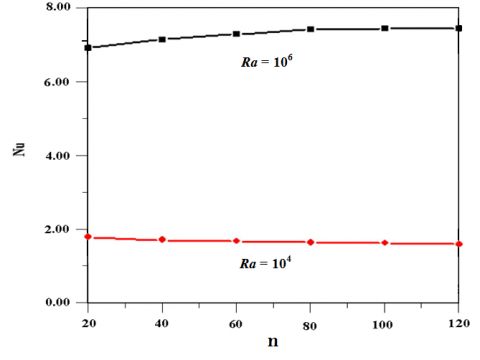Figure 2. Effect of the grid density on the average Nusselt number with AR = 0.4 and f = 0.00.

## 3.png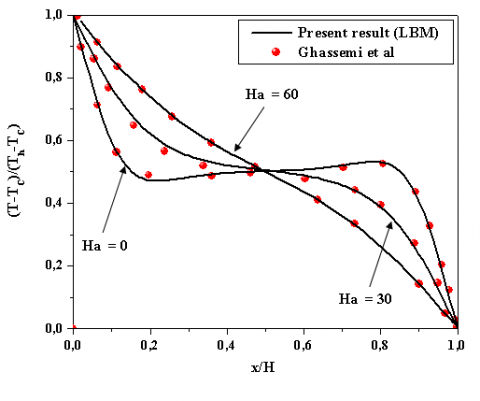Figure 3. Comparison of the temperature on axial midline between the present results and numerical results by Ghassemi et al.  (f = 0.03, Ra=105).

For validation of the written computer code, the present numerical model was validated at the too topics of this previous problem. The comparison of the temperature on axial midline for different values of the Hartmann number (Ha= 0, 30, 60), and for a solid volume fraction f = 0.03 showed a very good agreement with those of Ghasemi et al  for Ra = 105, (Figure 3). Based on the aforementioned comparisons, the developed code is reliable for studying MHD natural convection of a nanofluid confined in an L-shaped enclosure.

6. Results and Discussion

Numerical analysis of buoyancy induced flow due to both the thermal effect in an inclined L-shaped enclosure filled with water based nanofluid has been performed. The effects of aspect ratio (AR), volume fraction of nanoparticles (f), Rayleigh number (Ra) and Hartmann number (Ha), are discussed, in the cavity in terms of streamlines and isotherms, local and average Nusselt numbers. Comparison of two cases as absence of Brownian effect and presence of Brownian effect will be discussed.

6.1. Effects of Rayleigh and aspect ratio AR

Figure 4 shows the pressure contours of nanofluid (f = 0.04) for Ra = 104 at different aspect ratio (AR). It is found from this figure that there are one area of unstable region in the domain (close to the top wall). This phenomenon is explained as follows. As the boundary conditions are defined, the temperature on the left wall is lower than the temperature of the fluid, which leads the fluid with higher temperature in the cavity to move upwards. Also, the pressure difference develops gradually due to the movement of the fluid in the cavity, and the irregular phenomena of the total pressure contours indicate that the flow of the fluid is unstable. Consequently, when the heated fluid expands and moves upwards to the top wall, the pressure close to the top wall is much higher than any other area. A similar interpretation for Figure 5 illustrates the effect of the L-shaped enclosure aspect ratio (AR = H/D) on the isotherms, streamlines and pressure contours of nanofluid (f = 0.04) for Ra = 104 and 106. As can be seen from the isotherms in this figure, for Ra = 104 and for all aspect ratio, the isotherms are parallel to the enclosure walls that implies a conduction heat transfer regime in the cavity. Hence, vortex with elliptical core is formed in the vertical part of the cavity. The maximum stream function magnitude occurs in the central core of the L-shaped enclosure. On the other hand, the streamlines, do not take place under the cold rib. It is because of existence of small gap between the hot bottom wall and the cold rib which limits the flow movement, especially for AR=0.2. But, increasing the Ra number from 104 to 106, the isotherms are not parallel to the walls and thermal boundary layers are formed near the vertical walls. This is because the buoyancy force effect is important, so the convection heat transfer contribution is important too and the conduction heat transfer is neglected. Also, as can be seen from the streamlines in this figure, for AR=0.2, the fluid is heated by the hot walls (AB and BC) and expands as it moves upward. Then the fluid is cooled by the cold walls (DE and EF) and compressed as it moves downward. In the vertical part of the cavity just one clockwise eddy is established but five eddies are formed and rotating in opposing directions in the other part of the cavity. At AR = 0.3 and 0.4, three vortexes are formed in the horizontal part of the L-shaped enclosure. Further increasing the AR to 0.5, the three vortexes in the horizontal part coalesce to make a larger and stronger counter-clockwise vortex. On the other hand, it is shown that the maximum value of the stream function increases with the rise of aspect ratio for all Rayleigh numbers. Also the effect of aspect ratio is not identical for different Rayleigh numbers. For instance, from AR=0.2 to 0.5 the values of the maximum stream function decrease by 93% and 56% for Rayleigh numbers of Ra=104 and 106 respectively.

## 4.png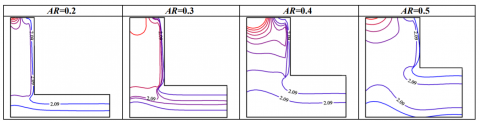Figure 4.  Pressure contours of nanofluid (f = 0.04) for Ra = 104 at different aspect ratio (AR). (Al2O3-H2O nanofluid).

## 5a.png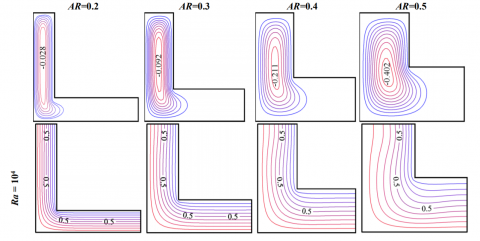## 5b.pngFigure 5. Isotherms and streamlines for different Ra and AR at f = 0.04 (Al2O3-H2O nanofluid).

Table 2 presents a comparison study for the increase in the average Nusselt number for the nanofluid at various aspect ratios (0.2≤AR≤0.5) and solid volume fractions (0≤f≤0.04) for Ra=104 when Brownian motion is considered. The results show that the effect of the aspect ratio of the enclosure has a significant influence on heat transfer, depending on the solid volume fraction. As it can be seen from this table, the maximum effect occurs at AR = 0.2. For example, from f = 0.0 to 0.04 the values of the average Nusselt numbers decrease by 21.94% and 12.76% for aspect ratios numbers of AR =0.2 and 0.5 respectively. This can be explained by the fact that the accumulation of the nanoparticules in small region caused a favorable enhancement of the heat transfer

Table 2. The Brownian motion effects on Num various AR and f, at Ra=104

 Ra=104 f=0.00 f=0.04 Ar= 0.2 4.31 5.256 Ar= 0.3 2.64 3.218 Ar= 0.4 1.884 2.24 Ar= 0.5 1.551 1.749

6.2 Effects of the Hartmann number

Figure 6 illustrates the influence of the magnetic field on the streamlines and isotherm contours on nanofluid (f = 0.04) with AR = 0.4, for different values of the Hartmann number (Ha= 0, 20, 40 and 60) and for Ra=104 and 106. The magnetic field reduces the heat transfer and fluid circulation within the enclosure due to the retardation effect of the electromagnetic body force. This is accompanied with significant reduction in the fluid movement in the cavity. For Ra=104, the retardation effect of the magnetic effect is noticed from the maximum strength of circulation |ψ|max. The strength of streamlines is decreased by Hartmann number, while the maximum value of streamlines when the Brownian motion of nanoparticle is considered in the enclosure is equal to 0.211 for Ha=0.0, 0. 95 for Ha =20, 0.042 for Ha =40 and 0.024 for Ha =60. Further increasing the Ra to 106, it is noticeable that the three vortexes in the horizontal part coalesce to make a larger counter-clockwise vortex. The maximum strength of this circulation |ψ|max is decreased by Hartmann number, is equal to 0.582 for Ha= 20, 0.384 for Ha =40 and 0.284 for Ha =60. Finally, the effect of magnetic field on the isotherms is noticed by the gradual shifting of the contours to the vertical distribution in the core region of the enclosure. As the Hartmann number increases a reduction on the temperature gradients near the cavity wall occurs. This is an indication for the approach of the quasi-conduction regime within L-shaped enclosure.

## 6a.png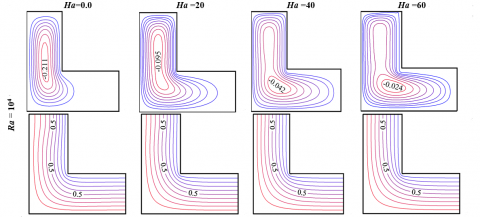## 6b.png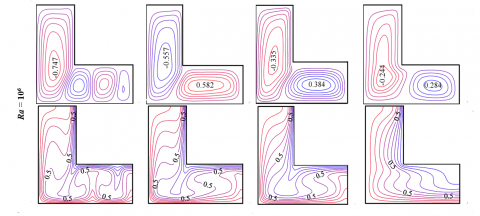Figure 6. Isotherms and streamlines for different Ra and Ha at f  = 0.04 (Al2O3-H2O nanofluid).

Existence of the nanoparticles in the water changes the properties of the base fluid and hence affects the heat transfer and fluid flow characteristics. The presence of high thermal conductive nanoparticles enhances the thermal conductivity of the nanofluids and causes a favorable enhancement of the heat transfer. On the other hand, the increase of nanofluid viscosity decreases convection heat transfer. So, this last effect of nanoparticles is unfavorable from the point of view of heat transfer. Figure 7, present the Brownian motion effect on the variation of average Nusselt number for, Ha= 0, AR=0.2 and different Rayleigh numbers (Ra=104, 106). A comparison between the two studies of with and without Brownian motion shows that the heat transfer is generally higher when Brownian motion is considered. This can be explained due the fact that when the Brownian motion is considered, the thermal conductivity by micro-scale convective heat transfer of a particle’s Brownian motion is enhanced. At the first part, for all Rayleigh number, when Brownian motion is neglected, the average Nusselt number continuously increases as f  increases. However, for Ra =104 when Brownian motion is considered, an optimum value for f can be found which results in the maximum Num. At Ra=106, the Brownian motion effect acts as an effective parameter to augment heat transfer when f < 0.02. On contrast, when the solid volume fraction increase from f= 0.02 to 0.04, the average Nusselt number decreases. Therefore, the Brownian motion effect on the enhancement appears at low volume fraction of the nanoparticles. So, the favorable enhancement of the nanofluid has a dominant effect on the thermal conductivity, it leads to enhancement of the Nusselt numbers, but the unfavorable increase of the nanofluid viscosity affects on some amount this enhancement. But, on the contrary at high values of the solid volume fraction f > 0.02, the Brownian motion of the nanoparticles has an adverse effect on heat transfer. This is due to the fact that nanofluid becomes more viscous which will reduce convection currents and accordingly will diminish the Nusselt number and consequently the temperature at the heated surface. Figure 8, presents the influence of the Hartman number (Ha) and nanoparticles volume fraction (f) on the average Nusselt number along the heated surface for different Rayleigh numbers (Ra=104, 106). It demonstrates that the average Nusselt number is raised steadily non-linearly by the augmentation of the nanoparticles volume fraction at various Hartmann and Rayleigh numbers. It can be seen from these figures that the magnetic field effect is to suppress the heat transfer within the cavity by decreasing the average Nusselt number. Also, it can be seen from these figures that the effect of magnetic field on Nusselt number is more significant at high Rayleigh numbers (Ra=106). For instance, at Ra=103 and f= 0.04, the difference between Num (Ha=0.0) and Num (Ha=60.0) is about 4%, while it is increased by 41% for Ra=106. On the other hand, the results show that the effect of Hartman number is vanished at law Rayleigh numbers (Ra=104), when Ha> 40.

## 7.png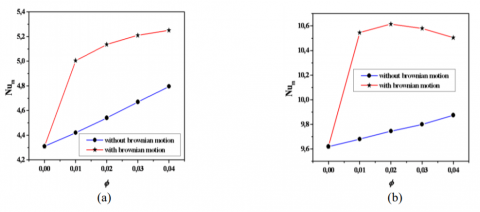Figure 7. Average Nusselt number on the left wall for different Ra and f  , (a) Ra=104, (b) Ra=106 .

## 8.pngFigure 8. Average Nusselt number on the left wall for different Ha and f  , (a) Ra=104, (b) Ra=106.

7. Conclusions

In this study, MHD natural convection heat transfer in an inclined L-shaped enclosure filled by Al2O3-H2O nanofluid is investigated numerically using the Lattice Boltzmann Method. The effects of nanoparticles volume fraction, aspect ratio and Rayleigh numbers on the flow and heat transfer characteristics have been examined. The following main conclusions can be made according to the numerical results:

• The maximum stream function values increase with the Rayleigh number and aspect ratio of the cavity.
• For all Ra and AR, the enhancement in heat transfer is observed when Brownian motion is considered.
• The aspect ratio of the cavity has significant effect on the velocity and temperature fields.
• Transition point from conduction to convection heat transfer mode is postponed at higher Ra number 106, especially for AR =0.2.
• The magnetic field reduces the heat transfer and the fluid flow intensity.
• Further increase in Ha numbers than 40, had a negligible effect on the average Nusselt number at Ra = 104.
Nomenclature
 B dp Ha                   k Ma                   Nu P                    Pr                      Ra                     T             $\mathbf{u}(u, v)$            $\mathbf{x}(x, y)$ H Greek symbols α β kb Δx Δt f µ $\rho$ $\theta$ V  σ Subscript c f h nf p Magnetic field, Tesla = N/A.m2 diameter of particle, nm Hartmann number Thermal conductivity, W/m.K Mach number Local Nusselt number Pressure, N/m2 Prandtl number Rayleigh number Temperature, K Velocities, m/s Lattice coordinates, m height of cavity, m   Thermal diffusivity, m2/s Coefficient of thermal expansion, K-1 Boltzmann constant, J. K-1 Lattice spacing Time increment Solid volume fraction Dynamic viscosity, kg/m.s Fluid density , kg/m3 Non-dimensional temperature Kinematic viscosity, m2/s Electrical conductivity, (Ω.m)-1   cold surface fluid hot surface nanofluid particle
References

 D. Yadav, D. Nam and J. Lee, “The onset of transient Soret-driven MHD convection confined within a Hele-Shaw cell with nanoparticles suspension,” J Taiwan Inst Chem Eng, vol. 58, pp. 235–244, 2016.

 Y. Dhananjay, R. Bhargava and G.S. Agrawal, “Thermal instability in a nanofluid layer with vertical magnetic field,” J Engineering Mathematics, vol. 80, pp. 147–164, 2013.

 D. Yadav, R. Bhargava, G.S. Agrawal, G.S. Hwang and J. Lee, M.C. Kim, “Magneto-convection in a rotating layer of nanofluid,” Asia-Pacific J Chemical Engineering , vol. 9, pp. 663–677, 2014.

 D. Yadav, C. Kim, J. Lee and H.H. Cho, “Influence of magnetic field on the onset of nanofluid convection induced by purely internal heating,” Computers and Fluids, vol. 121, pp. 26–36, 2015.

 S.K. Das, N. Putra and W. Roetzel, “Pool boiling characterization of nano-fluids,” Int J Heat Mass Tranf, vol. 46, pp. 851-862, 2003.

 B. Mliki, M.A. Abbassi and A. Omri, “Lattice Boltzmann simulation of natural convection in an L-shaped enclosure in the presence of nanofluid,” Engineering Science and Technology, an International Journal, vol. 18 pp. 503–511,2015.

 H.M. Elshehabey, F.M. Hady, Sameh E. Ahmed and R.A. Mohamed, “Numerical investigation for natural convection of a nanofluid in an inclined L-shaped cavity in the presence of an inclined magnetic field,” Int. Commun. Heat Mass Transf, vol. 57, pp. 228–238, 2014.

 B. Mliki, M.A. Abbassi and A. Omri, “Lattice Boltzmann simulation of MHD double dispersion natural convection in a c-shaped enclosure in the presence of a nanofluid,” Fluid Dynamic and Material Processing,  vol. 11, no. 1, pp. 87-114, 2015.

 L. Chin and C. Cheng, “Numerical predictions of natural convection with liquid fluids contained in an inclined arc-shaped enclosure,” Int Commun Heat Mass Transfer, vol. 39, pp. 209–215, 2012.

 M. Mahmoodi and S.M. Hashemi, “Numerical study of natural convection of a nanofluid in C-shaped enclosures,” Int J Therm Sci, vol. 55, pp. 76-89, 2012.

 M. Saidi and G. Karimi, “Free convection cooling in modified L-shape enclosures using copper-water nanofluid,” Energy, vol. 70, pp. 251-271, 2014.

 N. Makulati, A. Kasaeipoor and M.M. Rashidi, “Numerical study of natural convection of a water–alumina nanofluid in inclined C-shaped enclosures under the effect of magnetic field,” Advanced Powder Technology, vol. 27, pp. 661–672, 2016.

 M.A. Mansour, A.Y. Bakier and M.A.Y. Bakier, “Natural convection of the localized heat sources of T-shaped nanofluid-filled enclosures,” Am. J. Eng. Res, vol. 2, pp. 49–61, 2013.

 B. Ghasemi and S.M. Aminossadati, “Brownian motion of nanoparticles in a triangular enclosure with natural convection,” Int. J. Therm. Sci, vol. 49, pp. 931-940, 2010.

 Z. Haddad, E. A. Nada, H. F. Oztop and A. Mataoui, “Natural convection in nanofluids: Are the thermophoresis and Brownian motion effects significant in nanofluid heat transfer enhancement,” Int. J. Therm. Sci, vol. 57, pp. 152–162, 2012

 B. Mliki, M. A. Abbassi, A. Omri and B. Zeghmati, “Effects of nanoparticles Brownian motion in a linearly/sinusoidally heated cavity with MHD natural convection in the presence of uniform heat generation/absorption,” Powder Technol, vol. 295, pp. 69–83, 2016.

 J. Koo and C. Kleinstreuer, “A new thermal conductivity model for nanofluids,” J Nanoparticle Research, vol. 6, pp. 577-588, 2004.

 H.C. Brinkman, “The viscosity of concentrated suspensions and solution,” J Chemical Physics, vol. 20, pp. 571-581, 1952.

 M. Sheikholeslami, M. Gorji-Bandpy, R. Ellahi and A. Zeeshan, “Simulation of MHD CuO–water nanofluid flow and convective heat transfer considering Lorentz forces,” J Magn Magn. Mater, vol. 369, pp. 69–80, 2014.

 A. Mahmoudi, I. Mejri, M. A. Abbassi and A. Omri, “Magnetic Field Effect on Natural Convection in a nanofluidfilled enclosure with non-uniform heating on both Side Walls,” International Journal of Heat and Technology, vol. 32, no. 1, pp. 127-133, 2014.

 A. Mahmoudi, I. Mejri and A. Omri, “Study of natural convection in a square cavity filled with nanofluid and subjected to a magnetic field,” International Journal of Heat and Technology, vol. 34, no. 1, pp. 73-79, 2016.